Project
Project ref
Calcs for
Date
To Eurocode BS EN 1993-1-1/NA:2008

## Design summary

 Resistance / Limit Applied / Actual Utilisation Shear 61.3 kN 10.7 kN 18 % OK Bending moment 6.71 kNm 2.69 kNm 40 % OK Buckling 5.12 kNm 2.69 kNm 52 % OK Total deflection 5 mm 1.5 mm 29 % OK Deflection due to variable actions 2.8 mm 0.6 mm 21 % OK

## Section details

 Type Parallel flange I section Section IPE AA 80 Steel grade S355 Width b = 46 mm Depth h = 78 mm Web thickness tw = 3.2 mm Flange thickness tf = 4.2 mm Root radius r = 5 mm Mass per metre w = 4.9 kg/m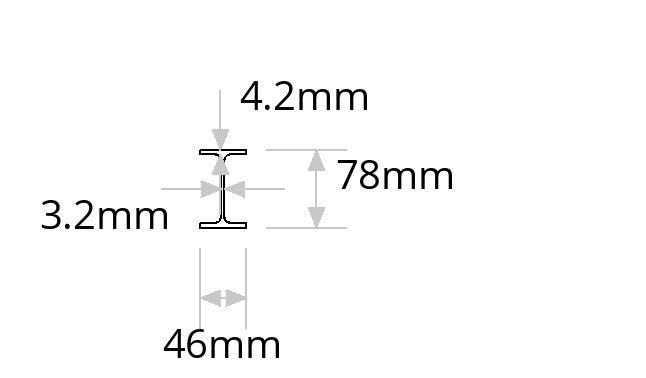## Span and restraints

 Effective span L = 1,000 mm Buckling length Lcr = 1,000 mm

## Deflection limits

 Variable action deflection limit ΔQ = L / 360 = 2.78 mm Total deflection limit ΔG+Q = L / 200 = 5 mm

## Safety factors

 Partial factor for permanent actions γG = 1.35 Partial factor for variable actions γQ = 1.5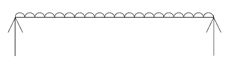Self weight
 Permanent action SW = w × 9.81 / 1000 = 0.0481 kN/mLoad 1: UDL - Sloping roof, 0° to 30°
 Permanent action G1 = 1.15 kN/m² × 8 m = 9.2 kN/m Variable action Q1 = 0.75 kN/m² × 8 m = 6 kN/m

## Reactions

 Permanent (unfactored) Variable (unfactored) Total (unfactored) Total (factored) Left reaction 4.62 kN 3 kN 7.62 kN 10.7 kN Right reaction 4.62 kN 3 kN 7.62 kN 10.7 kN

## Design shear force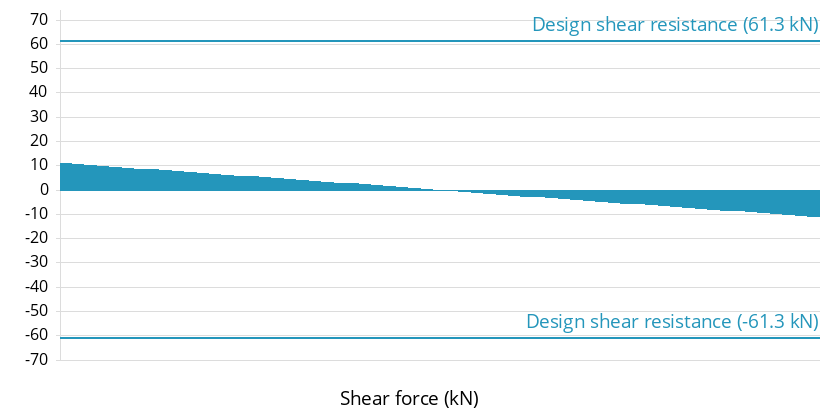Design shear force VEd = 10.7 kN Design shear resistance Vc,Rd = 61.3 kN Utilisation VEd / Vc,Rd = 18 % OK

## Design bending moment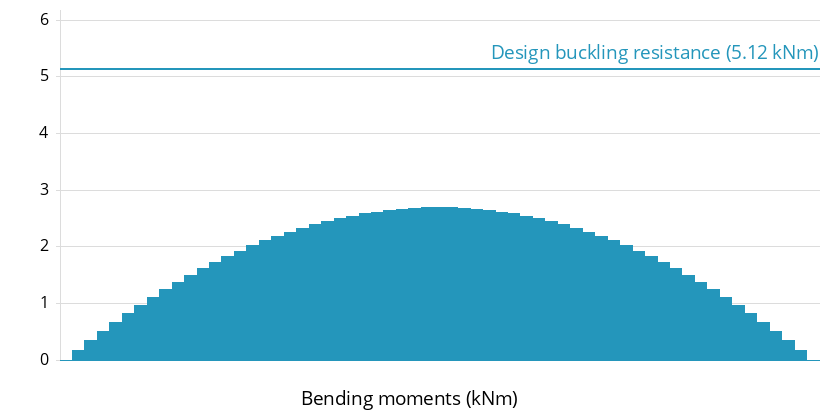Design bending moment, major axis MEd = 2.69 kNm Design resistance for bending Mc,Rd = 6.71 kNm Bending utilisation MEd / Mc,Rd = 40 % OK Design resistance for buckling Mb,Rd = 5.12 kNm Buckling utilisation MEd / Mb,Rd = 52 % OK

## Deflection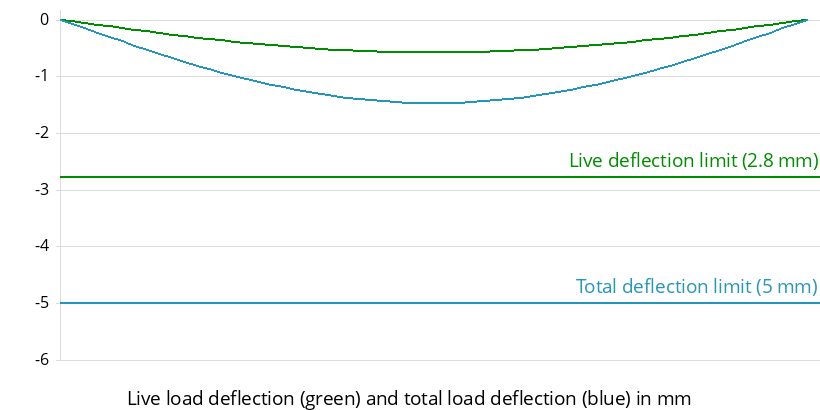Variable action deflection limit ΔQ = 2.8 mm Variable action deflection δQ = 0.6 mm OK Total deflection limit ΔG+Q = 5 mm Total deflection δG+Q = 1.5 mm OK

### Section properties

Elastic modulus - major axis, yy Wel = 16.4 cm3
Plastic modulus - major axis, yy Wpl = 18.9 cm3
Second moment of area - major axis, yy Iy = 64.1 cm4
Second moment of area - minor axis, zz Iz = 6.85 cm4
Warping constant Iw = 0.00009 dm6
Torsional constant IT = 0.38 cm4
Area of section A = 630 mm2

### Factors and design values of material coefficients (EN 1993-1-1:2005 and National Annex)

Young's modulus of elasticityE = 210,000 N/mm²cl.3.2.6
Poisson's ratio in elastic stagev = 0.3 cl.3.2.6
Shear modulusGs = 81,000 N/mm²cl.3.2.6
Partial factor for resistance of cross-sectionsγM0 = 1 cl.6.1(1)B / BS-EN NA
Partial factor for resistance to instabilityγM1 = 1 cl.6.1(1)B / BS-EN NA
Factor for shear areaη = 1 EN 1993-1-5:2006 cl.5.1(2) / BS-EN NA
Limiting non dimensional slenderness ratioλLT,0 = 0.4 cl.6.3.2.3(1) / BS-EN NA
Beta factor for buckling reduction factor calculationβ = 0.75 cl.6.3.2.3(1) / BS-EN NA

### Yield strength

Nominal yield strength for S355 grade and nominal section thickness 4.20 mmfy = 355 N/mm²Arcelor Mittal orange book

### Section classification (EN 1993-1-1:2005 cl.5.5)

Epsilonε = 0.814EN 1993-1-1:2005 Table 5.2
Flange ratio for local bucklingcf / tf = 3.9
Flange ratio limit for class 19 ε = 7.32Table 5.2 (sheet 2 of 3)
Flange classClassf = 1
Web ratio for local bucklingcw / tw = 18.6
Web ratio limit for class 172 ε = 58.6Table 5.2 (sheet 1 of 3)
Web classClassw = 1
Section classClass = 1

### Shear resistance (EN 1993-1-1:2005 cl.6.2.6)

Height of webhw = 69.6 mm
Shear area for I and H sectionsAv = 299 mm²cl.6.2.6 (3)
Design shear resistanceVpl,Rd = 61.3 kNeq (6.18)

### Shear buckling (EN 1993-1-5:2006 cl.5)

The shear buckling resistance for webs should be verified according to Section 5 of EN 1993-1-5 if (hw / tw) > (72 ε / η)
Web ratio for shear bucklinghw / tw = 21.7 EN 1993-1-5:2006 cl.5.1 (2)
Shear buckling limit72 ε / η = 58.6 EN 1993-1-5:2006 cl.5.1 (2)
(hw / tw) <= (72 ε / η) therefore shear buckling calculation not required

### Bending resistance (EN 1993-1-1:2005 cl.6.2.5)

The shear force (11 kN) is less than half of the plastic shear resistance (61 kN / 2 = 31 kN), therefore its effect on moment resistance may be neglected.
Class 1 section, therefore use plastic modulusWpl = 18,900 mm³
Design bending resistanceMc,Rd = 6.71 kNmeq (6.13)

### Design buckling resistance (EN 1993-1-1:2005 cl.6.3.2)

C1 factorC1 = 1
Shear modulus of elasticityGs = 81,000 N/mm²cl.3.2.6 (1)
Buckling lengthLcr = 1,000 mm
Critical buckling momentMCR = 8.38 kNmNCCI SN003b-EN-EU
Class 1 section, therefore use plastic modulusWpl = 18,900 mm³cl.6.3.2.1(3)
Non-dimensional slenderness ratioλLT = 0.895cl.6.3.2.2 (1)
Depth to width ratio for buckling curveh / b = 1.7
Buckling curve for h / b ratioBuckling curve = bTable 6.5 / BS-EN NA
Imperfection factor for buckling curve bαLT = 0.34Table 6.3 / BS-EN NA
Intermediate factor for reduction factor calculationφLT = 0.884cl.6.3.2.3 (1)
Buckling reduction factorχLT = 0.763eq (6.57)
Correction factor for moment distributionkc = 1Table 6.6
Moment distribution modification factorf = 1cl.6.3.2.3 (2)
Modified buckling reduction factorχLT,mod = 0.763eq (6.58)
Design buckling resistanceMb,Rd = 5.12 kNmeq (6.55)

## Notes

C1 value conservatively taken as 1.0

Ends of beam are to be laterally restrained. Ends of beams can be laterally restrained using one of the following methods;

1) End of beam built into masonry wall.
2) End of beam fixed to a masonry wall.
3) End of beam fixed to a column or a beam.

The designer is to ensure that the proposed detail adequately ensures that the end of the beam is laterally restrained.

No allowance has been made for destabilising loads which are outside the scope of these calculations (Destabilising loads would not normally occur in a traditional masonry structure)

DEMO